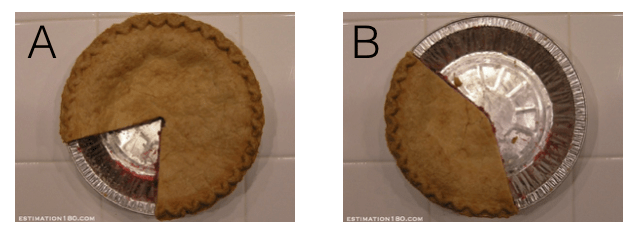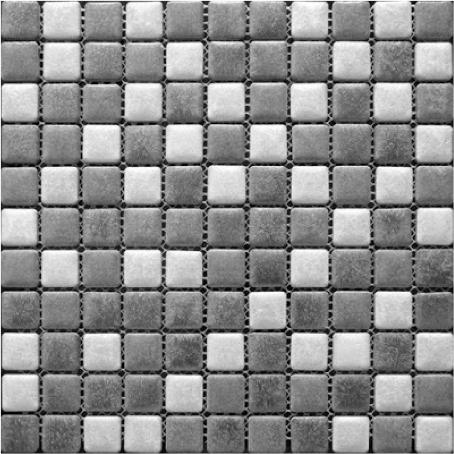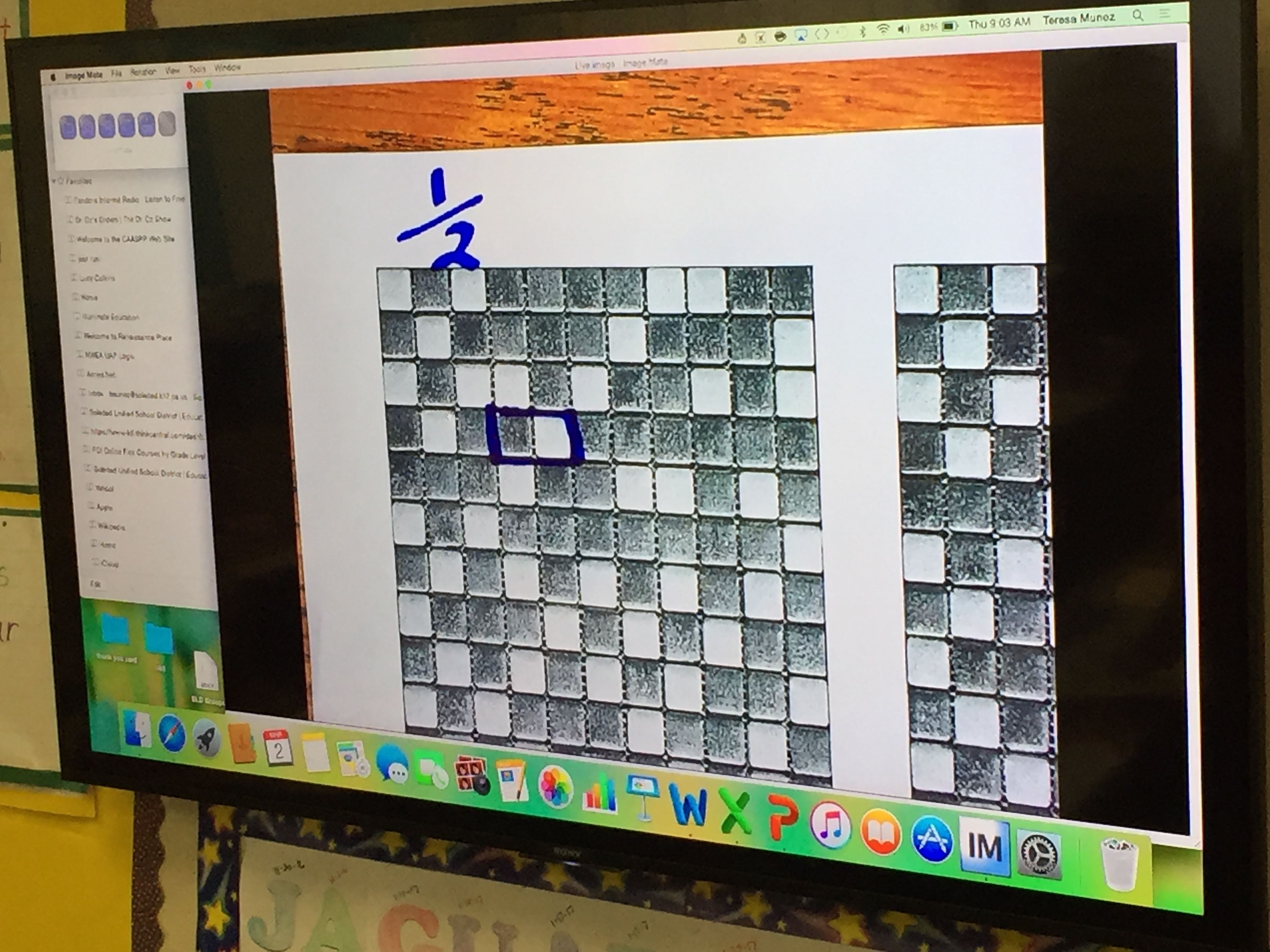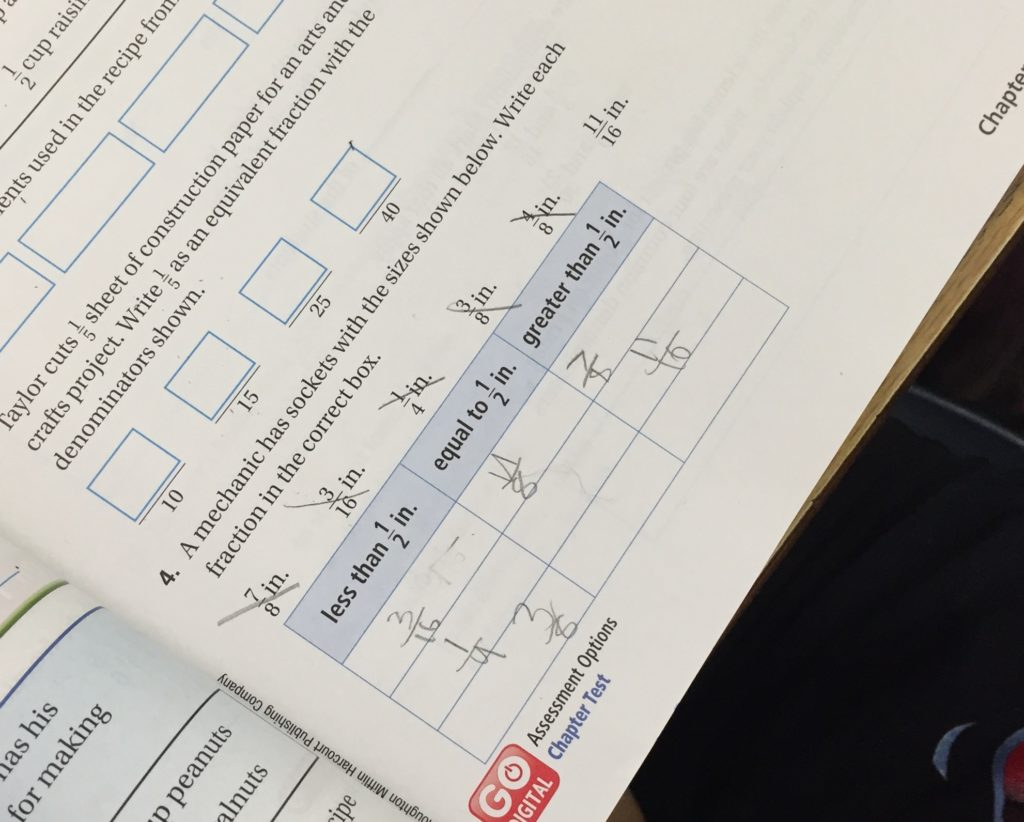# A Fraction Talk in 4th Grade

Have you seen the amazing visuals over at www.fractiontalks.com?  They’re ideal for any teacher looking to get all students, regardless of ability levels, talking about equivalent fractions (4.NF.1), comparing the value of fractions (4.NF.2), and expressing mathematical reasoning.  This activity could also work for 3rd grade students that are exploring fraction equivalence (3.NF.3).  We used this image to introduce 1/2 as a benchmark fraction to some 4th grade students.  (If you want to read about our 3rd grade experience, click here.)  We learned a lot and the students did too!  We’d like to share our learning with you.

I hope that there are other 4th (3rd and 5th) grade teachers out there that might find this analysis useful if they are looking for a low-floor introduction to comparing the value of fractions.  It also allows for rich discussion and sense-making because there are many possible representations for fractions that are equal to, less than, and greater than 1/2.  The lesson also allows students to use visual models to compare fractions using one half as a benchmark fraction.

Please feel free to plagiarize and make it your own as you see fit.  I’d love to hear from you if you use it so we can get better together.   You can find a copy of the handouts we used here and here.  (Special thanks to Nat Banting for curating a bank of such an amazing fraction visuals.  Check out the one we used here.  And a hat tip to Andrew Stadel for his sensational Estimation180 activities.  We used this one and this one.)

## Some quick background:

I conducted a lesson inquiry using these visuals with a team of 4th grade teachers.  Each lesson was a unique experiences with its own twists and turns and choices.  The lesson pathway below shows the main flow of the learning experience and took about 60 minutes.  Further analysis and takeaways can be found at the end of this post, but I’ve tried to embed a lot of our thinking within the flow of the lesson.

This lesson was taught to 2 classrooms of 4th grade students.  Students had a varying degree of skills comparing the value of fractions.  We used this lesson as a way to introduce the concept of a benchmark fraction using area models and describing colors in rectangles.

## Our objectives (as teachers):

• To make fractions meaningful and help students identify as learners, readers, and mathematical do-ers.
• To inspire students to interact and cooperate on learning opportunities.

## Learning objectives (for students):

• Students will compare fractions (using a benchmark fraction of ½).

## Into:

Note:  We did not focus on the objective at the start of the lesson.  We used the Into portion of the lesson as an opportunity for students to internalize the objective.  We did not refer to the objective until we were ready to conclude the Into and begin the Through part of the lesson.

To begin today’s lesson, please look at the TWO images on the board.  You have a black-and-white copy of this at your desks.What do you notice about these pictures?

Students had a lot to say.  We wanted them to make sure that they knew that the pictures are of pies and some pie is missing from each image.  We also wanted them to notice that image A showed more pie than image B.

So you’re comparing parts of the whole pie.  Interesting.

I have one more question for you.  Look at the images.  For each image, is there more than half of the pie left or less than half the pie left (showing)?  Explain your reasoning.

We gave them more talk time to discuss.  Most students intuit that B has less than half of a pie remaining because if they draw a line through the middle of the image, what’s left doesn’t take up half the pie.  Image A clearly shows almost a whole pie so it’s more than 1/2.

You just talked about how to compare parts (or fractions) of a whole pie.   You told me (correctly) that image A shows more pie than image B.  We also used the fraction 1/2 as a tool because 1/2 is such an easy fraction to see in pictures.

We gave them a moment to rephrase this to each other in their own words.  This is fundamentally the objective of the lesson.  And we provided the students a chance to internalize the objective before having it expressed explicitly.

Pictures make it easier to see the value of fractions.  Pictures make it easy to see if parts or a whole are equal or if one part is bigger than another.

It can be harder to compare things if we only have the fractions, the numbers.  But if we don’t have a picture, we can still determine if a fraction is less than, greater than, or equal to a half.  And that’s what we’re going to practice today.

YES!

## Through (part 1):

We displayed this image below from the fraction talks website, but we made it black and white so it looked like this:We wanted to make the image black and white to limit the information students had to process (only two colors instead of three in the original).  We also wanted to be able to print up lots of pictures for students to draw on and it made sense to go black and white.I’ve drawn a rectangle that is half white.  I want you to draw a rectangle that is also half white, but I challenge you to draw a rectangle that is bigger than mine!  Try to come up with a rectangle that no one else will come up with.  Remember, you need to show why you know your rectangle is half white.We monitored the room to see what students would do.  We wanted to see how well students could use fractions to describe their rectangles.  Some students kept verbally describing the reciprocal of fraction.  So after a few moments of work time, we scribed the structure “white/whole” on the board, paused the class, and had students who were using the structure explain why it’s useful.

If you’ve found a rectangle that is half white, try to find the largest one you can.  Use this fraction “white/whole” to describe each rectangle you draw.

This prompt is open and differentiates for individual student abilities.  Struggling students found rectangles that were 2/4 and 3/6 white.  Stronger students were off nerding out trying find the largest one they could.  It had a nice “open middle” feel to it.

Once all students had used a fraction to describe one of their rectangles, it was time for whole-class facilitation.

Can I have someone share one of the fractions?  Who else got this fraction?  Did anyone get a different fraction?

I scribed their fractions on the board.  As a whole class, your students should be able to find examples 1/2, 2/4, 3/6, 4/8, and 5/10.

(Note:  We anticipated getting some wrong answers, but we didn’t.  If we had, we were ready with:  Look at all these fractions.  You’re telling me they all equal a half.  Do any of them look like imposters?  Are there any that might not belong in this group?)

Great work.  Now remember, all of these fractions represent rectangles that are a half white.  Therefore, all of these fractions have a value of 1/2.

What do you notice about all of these fractions?

After some small group discussion, we asked groups to share their thinking.

Here are three things we heard in both classes:

• The numerator (top number) is half of the denominator (bottom number).
• The denominator is double/twice the numerator.
• If you add the numerator to itself, you get the denominator.

We scribed these clearly on the board.  We stressed both formal and informal vocabulary to keep the lesson accessible for all learners.

Come up with another fraction that you think equals one half.  Share them with others in your group.  Try to come up with a fraction no one else will.

We got some crazy responses involving really large numbers (like 50,000/100,000).  We shared and discussed a few examples as a whole class.  Students love to talk about their work when we give them open prompts and challenges that encourages them to be unique!

We made sure that all examples connected to the rules they had come up with previously (the bullet points above).

We concluded this part of the lesson by informally introducing the idea of a “benchmark fraction.”

Benchmark fractions are fractions that we really understand well in pictures and in number form.  One-half is probably the most important benchmark fraction.

We kept the language simple and easy and informal.  We planned to formalize language in future lessons.

## Through (part 2):

I then drew a rectangle that was more than half white and displayed it for the class to see.

Billy thinks that this rectangle is one half white.  Do you agree or disagree?  Why?

Brief discussion.  Again, we connected back to their bulleted list from before about the rules for fractions equivalent to 1/2 (Math Practice 3).

Is Billy’s rectangle more than half or less than half white?

More than half!

How do you know?

We had students discuss in groups before sharing with the class.  Most students saw that the numerator was more than half of the denominator.

New challenge!  Try to find rectangles that are more than half white.  Use fractions to describe them and explain how you know they are more than half white.

At this point, it may be useful to see what our board looked like near the end of this lesson.You’ll notice that I created a three column table and recorded class examples.  At this point in the lesson, we started listing examples in the right column.  We examined how we knew these fractions were greater than a half.  Students had the visual if they needed it, but we were encouraging them to make sense of the value of the fraction without the picture.  We reminded students that this was the objective of the lesson.

Ricardo said that he found a rectangle that was more than half white.  He wrote the fraction 2/2.  Has Ricardo made a mistake?  Is such a rectangle possible?

We gave some students some work time.  This question allowed students to make contextual connections between the picture and the numbers (Math Practice 2).  Ricardo’s rectangle is all white.  His fraction has a value of 1 because all of the parts are white.

We continued the lesson by asking students:

Now find a rectangle that is less than half white.  Use a fraction to describe it.

Group discussion time.  Then recording and discussing examples at the board.

Wanda found a rectangle that was 0/6th white.  What do we know about her rectangle?

Like Ricardo’s question, this prompt helps students contextualize the numbers with the pictures.  Wanda’s rectangle has a size of 6 and none of it is white.

## Beyond:

With this table completed and posted for students to use, we asked students to start comparing fractions more formally.  We only used fractions they had come up with and we only compared fractions that were in different columns.  For example, we did NOT ask students to compare 5/9 and 6/8.

We used questions like this:

Barbara drew a rectangle and says that 5/9 of the squares are white.  How does her rectangle compare to a rectangle that is ½ white?

As the prompt was given, we scribed the fractions on the board (but not the words).  We then let students have some work time and then discussed.  We encouraged them to only use the table of examples on the board if they needed to, but some students needed to find examples in the visual.  We then used < and > to compare the fractions.

We chose a few more examples to practice using similar language with rectangles.  For each example, we scribed the fractions on the board and used inequalities to show which fraction was greater.

Gina drew a rectangle that was 4/6 white.  Hugo drew a rectangle that was 1/5 white.  Which rectangle is more white?

We asked students to explain their reasoning using 1/2 as a benchmark fraction.  Some students begin to see that if the top and the bottom numbers are really close together in value, the fraction is greater than 1/2.  If the top number is really small compared to the bottom number, then the fraction is probably less than 1/2.

In one class, we were able to draw that language out and scribe it on chart paper for later use.  We didn’t quite get there in the other class and hope to have this understanding flushed out more in next lesson.

We concluded the lesson by giving them this problem to complete individually as an exit ticket.  It’s from their GoMath textbook and it’s a dreadfully pointless word problem.  We encouraged students not to be concerned about the word problem.  Instead, we showed how their table was like our table on the board and that their goal was to figure out the column in which each fraction belonged.

Where should the fractions go?## Reflections, Takeaways, Analysis:

At the end of the day, we reflected on our objectives and student learning.  Some things we noticed and wondered and a few professional goals moving forward:

• It’s important to let kids explore and find their own examples.  Students respond positively to open prompts.
• This tasks was appropriately challenging; it hit the sweet spot between accessibility and challenge for almost all students.
• Showing the visuals increased student interest and engagement compared to working out of the textbook.
• The numberless introduction (Andrew’s pie images) was low-floor so all students were on board right from the start.
• It’s important to do tasks like these.  The textbook leads student thinking too much; it limits their choice and voice in the learning process.  These tasks allow for an exchange of ideas.

## An invitation:

As always, feedback and comments are welcome.  What inspired you?  What opportunities did we miss?

Help us get better together.

This site uses Akismet to reduce spam. Learn how your comment data is processed.